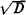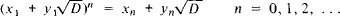# Pell's Equation

(redirected from Fermat's equation)
The following article is from The Great Soviet Encyclopedia (1979). It might be outdated or ideologically biased.

## Pell’s Equation

an equation of the form x2 - Dy2 = 1, where D is a positive integer that is not a perfect square and the equation is to be solved in integers. The equation has an infinite number of solutions. The solution x0 = 1, y0 = 0 is obvious. The next—in terms of magnitude—solution (x1, y1) of Pell’s equation can be found by expandinginto a continued fraction. If we know the solution (x1, y1), the entire set of solutions (xn yn) can be obtained by using the formulaPell’s equation is closely related to the theory of algebraic numbers. It is named after the 17th-century British mathematician J. Pell, to whom L. Euler incorrectly attributed a method of solving this equation.

### REFERENCES

Venkov, B. A. Elementarnaia teoriia chisel. Moscow-Leningrad, 1937. Chapter 2.
Dickson, L. E. History of the Theory of Numbers, vol. 2. New York, 1966.